##### Chemistry Workbook For Dummies with Online Practice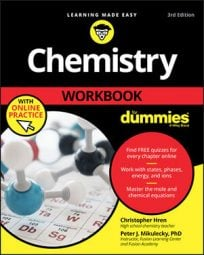To make it easier to work with extreme numbers, chemists turn to scientific notation, which is a special kind of exponential notation. A major benefit of presenting numbers in scientific notation is that it simplifies common arithmetic operations like multiplication and division.

## Multiplying in scientific notation

To multiply two numbers written in scientific notation, you work out the coefficients and the exponents separately. Here’s an example: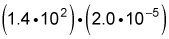To solve this problem, you simply follow these steps:

1. Multiply the coefficients.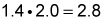2. Add the exponents of the powers of 10.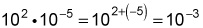3. Join the new coefficient to the new power of 10 to get the answer.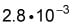## Dividing in scientific notation

To divide two numbers written in scientific notation, you also work out the coefficients and the exponents separately, but now it involves division and subtraction. Here’s an example: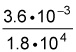To solve this problem, you follow these steps:

1. Divide the coefficients.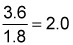2. Subtract the exponent in the denominator from the exponent in the numerator.

Remember that the denominator is the bottom number and the numerator is the top number.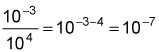3. Join the new coefficient to the new power of 10.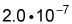Okay, time for a few practice questions.

## Practice questions

1. Multiply the following: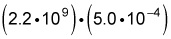2. Using scientific notation, divide the following: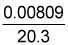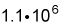First, multiply the coefficients: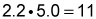Then multiply the powers of 10 by adding the exponents: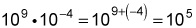The raw calculation yields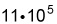which converts to the given answer when you express it in scientific notation: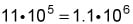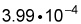First, convert each number to scientific notation: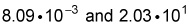Then divide the coefficients: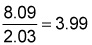Next, subtract the exponent on the denominator from the exponent of the numerator to get the new power of 10: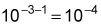Join the new coefficient with the new power:Finally, express gratitude that the answer is already conveniently expressed in scientific notation.